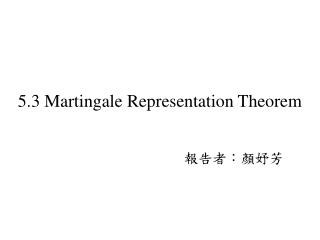DownloadDownload Presentation5.3 Martingale Representation Theorem

# 5.3 Martingale Representation Theorem

Télécharger la présentation## 5.3 Martingale Representation Theorem

- - - - - - - - - - - - - - - - - - - - - - - - - - - E N D - - - - - - - - - - - - - - - - - - - - - - - - - - -
##### Presentation Transcript

1. 5.3.1 Martingale Representation with One Brownian Motion

2. Corollary 5.3.2 is not a trivial consequence of the Martingale Representation Theorem , • Theorem 5.3.1, with replacing W(t) because the filtration in this corollary is generated by the process W(t), not the -Brownian motion . • The proof is left to the reader as Exercise5.5

3. 5.3.2 Hedging with One Stock • Now has the stock price process (5.2.15) and an interest rate process R(t) that generates the discount process(5.2.17) • Recall the assumption that, for all t [0,T], the volatility is almost surely not zero. • We make the additional assumption that the filtration , 0 t T, is generated by the Brownian motion W(t), 0 t T.

4. Let V(T) be an -measurable random variable and, for 0 t T, define V(t) by the risk-neutral pricing formula (5.2.31). Then, according to (5.2.30), • This is a - martingale; indeed, iterated conditioning implies that, for 0 s t T, (5.3.3)

5. Therefore, has a representation as (recall that ) • For any portfolio process , the differential of the discounted portfolio value is given by (5.2.27), and hence (5.3.5)

6. In order to have X(t)=V(t) for all t, we should choose X(0)=V(0) (5.3.6) • choose to satisfy (5.3.7) which is equivalent to (5.3.8) With these choices, we have a hedge for a short position in the derivative security with payoff V(T) at time T.

7. There are two key assumptions that make the hedge possible. • The first is that the volatility is not zero, so equation (5.3.7) can be solved for . • The second is that F(T) is generated by the underlying Brownian motion (i.e., there is no randomness in the derivative security apart from the Brownian motion randomness, which can be hedged by trading the stock). • Under these two assumptions, every F(T)-measurable derivative security can be hedged. Such a model is said to be complete.

8. Thanks for your listening !!